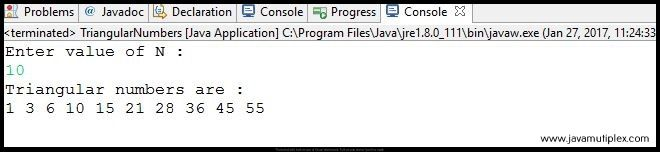## Problem Description :

Write a program in Java that generates first N triangular numbers.First N Triangular numbers

## Concept :

We can generate Nth triangular number or triangle number using below formula.
N(N+1)/2
For example - first 6 triangular numbers are 1, 3, 6, 10, 15, 21.

## Output :Output - How to generate first n triangular numbers in Java?

## References :

https://en.wikipedia.org/wiki/Triangular_number

http://www.9math.com/book/sum-first-n-natural-numbers

Thank you friends, I hope you have clearly understood the solution of this problem. If you have any doubt, suggestion or query please feel free to comment below. You can also discuss this solution in our forum.

Tags : Triangular numbers in Java, Solution in Java, Mathematics problems, for loop, if else statement, Sum of first n natural numbers.How to generate first N triangular numbers in Java?Reviewed by Rohit Agarwal on 1/27/2017 Rating: 5